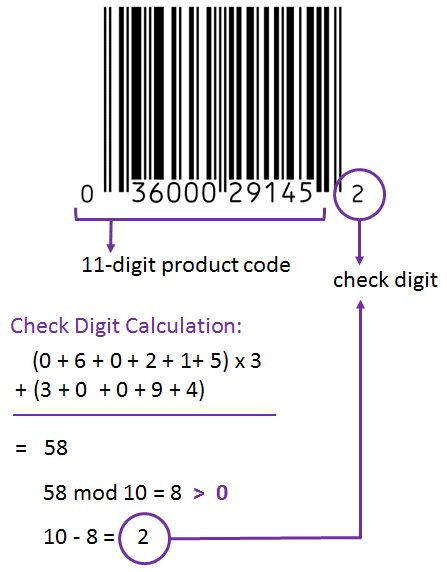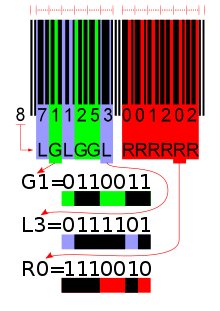### Symbology of the EAN code

The 13 digit EAN barcode is made up of a 12 digit string plus a check digit at the end. The 12 digits are a unique series that has never been assigned to a retail product before, and the last digits corrects for errors in scanning or data entry. There is a 5 step process used to calculate the check digit based off of the previous 12 digits:

1: Start from the end of the barcode number and add up every alternate digit. Do not include the check digit if it is already present. For example, if our barcode number is 0712345678911, we will remove the 1 at the end (because it is the check digit), and then add up every second number starting on the right side. So we will get 1+8+6+4+2+7 = 28

2: Multiply the result from the previous step by 3. 28 x 3 = 84

3: Add up all the remaining digits (excluding check digit again if present), so in our example this will be 0+1+3+5+7+9 = 25

4: Add the result from step 3 to the result from step 4. 84+25 = 109

5: The check digit is the smallest number which can be added to the result of step 4 to create a multiple of 10. So in our case, we can add 1 to 109 to make 110, a multiple of 10. This makes 1 our check digit.The black bars of the barcode encode the numbers represented underneath in a format that can be read by a digital scanner. Each number is represented by two black bars and two empty white spaces. The width of the bars and spaces is what differentiates the digits when the barcode is scanned. There are three different encoding schemes (different bar combinations for each digit 0-9), known as L, G and R. All EAN barcodes begin with an L encoded digit and end with an R encoded digit, which means that scanners can determine the beginning and end of a barcode and thus scan it upside down without issues.